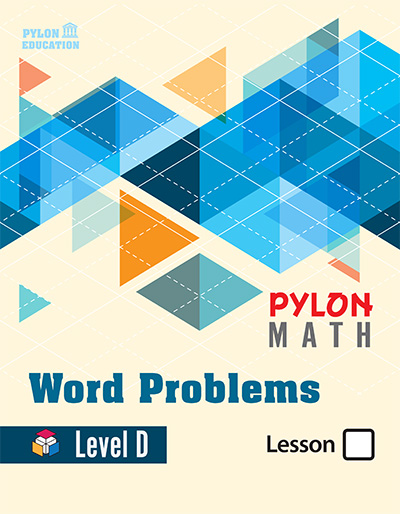# Word Problem Level D1. ### Place Values

• Ten-Thousands
• Hundred Thousands, Millions
• Roman Numerals
• Benchmark Numbers
• Compare Numbers, Order Numbers
• Round Numbers, Data Analysis
• Word Problems
2. ### Sum & Difference

• Subtraction, Mental Math
• Counting Money
• Word Problems
• Multiple Step Problems
• Sum or Difference, Estimation
• Critical Thinking
3. ### Expression and Elapsed Time

• Equations, Find a Rule
• Telling Time, Use Data
• Departure Time
4. ### Graphs and Properties

• Bar Graphs and Pictographs
• Line Graphs, Line Plots,
• Stem-and-Leaf Plots, Data Analysis
• Pictographs, Critical Thinking
• Properties, Order of Operations
• Expressions with Variables, Patterns
5. ### Multiplication

• Meaning of Multiplication
• Multiplying with 3, 4, 6, 7, 8
• Patterns in 9, 10, 11, and 12
• Decision Making, Critical Thinking
• Meaning of Division and Multiplication
• Even and Odd Numbers
• Factors, Problem Solving
1. ### Division

• Remainders
• Dividing with 2, 5, 9
• Special Quotients
• Dividing with 3, 4, 6, 7, 8
• Problem Solving Strategies
• Mental Math, Division Patterns
• Estimate Quotients
• Find the Mean
2. ### Divisibility Rules

• Divisibility Rules of 2, 3, 5, 9,10
• Factors of Multiples
• Prime and Composite Numbers
• Find a Pattern
• Square Numbers
• Decimals and Fractions
3. ### Geometric Figures

• Line Segments, Line, Ray, Plane
• Angles, Protractor
• Intersecting, Parallel, Perpendicular
• Names of Polygons
• Circles, Turns
• Line Symmetry, Congruent Figures
4. ### Metric Units and Weights

• Inches, Foot, Yard, Mile
• Equivalent Measures
• Comparing Measurements
• Gallons, Quarts, Pints, Cups
• Changing Units
• Pounds, Ounces, Tons
• Weights of Objects